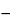C5H5N5 135.13
1H-Purin-6-amine;
1,6-Dihydro-6-iminopurine[73-24-5].
DEFINITION
Adenine contains NLT 98.0% and NMT 102.0% of C5H5N5, calculated on the dried basis.
IDENTIFICATION
ASSAY
•  Procedure
Blank:  80 mL of a mixture of 100 mL of glacial acetic acid and 300 mL of acetic anhydride
Titrimetric System
Mode:  Direct titration
Titrant:  0.1 N perchloric acid VS standardized as follows: Transfer 300 mg of potassium biphthalate to a 150-mL beaker and, by stirring, dissolve in 80 mL of a mixture of 100 mL of glacial acetic acid and 300 mL of acetic anhydride. Titrate with the perchloric acid solution. Each 20.42 mg of potassium biphthalate is equivalent to 1 mL of 0.1 N perchloric acid.
Endpoint detection:  Potentiometric
Analysis:  Dissolve the Sample in 80 mL of a mixture of 100 mL of glacial acetic acid and 300 mL of acetic anhydride by stirring, and titrate with the Titrant. Calculate the percentage of adenine (C5H5N5) in the portion taken:
Result = [(VB) × N × F × 100]/W
 V = = Sample titrant volume (mL) B = = Blank titrant volume (mL) N = = titrant normality (mEq/mL) F = = equivalency factor, 135.13 mg/mEq W = = weight of Sample (mg)
Acceptance criteria:  98.0%–102.0% on the dried basis
IMPURITIES
Organic Impurities
•  Procedure
pH 7.0 phosphate buffer:  Dissolve 4.54 g of monobasic potassium phosphate in water to make 500 mL of solution. Dissolve 4.73 g of anhydrous dibasic sodium phosphate in water to make 500 mL of solution. Mix 38.9 mL of the monobasic potassium phosphate solution with 61.1 mL of the dibasic sodium phosphate solution. Adjust, if necessary, by the dropwise addition of the dibasic sodium phosphate solution to a pH of 7.0.
Standard stock solution:  Dissolve a suitable quantity of USP Adenine RS in hot water, cool, and dilute quantitatively with water to obtain a solution having a known concentration of 0.19 mg/mL.
Standard solutions:  Pipet 5-mL portions of the Standard stock solution into three 100-mL volumetric flasks, and dilute with 0.10 N hydrochloric acid, 0.010 N sodium hydroxide, and pH 7.0 phosphate buffer, respectively, to volume.
Sample stock solution:  Dissolve a suitable quantity of Adenine in hot water, cool, and dilute quantitatively with water to obtain a solution having a known concentration of 0.19 mg/mL.
Sample solutions:  Pipet 5-mL portions of the Sample stock solution into three 100-mL volumetric flasks, and dilute with 0.10 N hydrochloric acid, 0.010 N sodium hydroxide, and pH 7.0 phosphate buffer, respectively, to volume.
Spectrometric conditions
Mode:  UV-Vis
Wavelegth range:  220–320 nm
Cell:  1 cm
Blank:  Water
Analysis
Samples:  Standard solutions and Sample solutions
Acceptance criteria:  The respective absorptivities, calculated on the dried basis, at the wavelengths of maximum absorbance, for each pair of corresponding solutions do not differ by more than 2.0%.
SPECIFIC TESTS
Dry a sample at 110for 4 h: it loses NMT 1.0% of its weight.
50.2%–53.4%, calculated on the dried basis